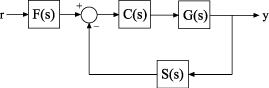Control System with Tunable Components

This example shows how to create a tunable model of the control system in the following illustration.The plant response is $G\left(s\right)=1/\left(s+1{\right)}^{2}$. The model of sensor dynamics is $S\left(s\right)=5/\left(s+4\right)$. The controller $C$ is a tunable PID controller, and the prefilter $F=a/\left(s+a\right)$ is a low-pass filter with one tunable parameter, $a$.

Create models representing the plant and sensor dynamics. Since the plant and sensor dynamics are fixed, represent them using numeric LTI models zpk and tf.

G = zpk([],[-1,-1],1);
S = tf(5,[1 4]);

Create a tunable representation of the controller $C$.

C = tunablePID('C','PID');

C is a tunablePID object, which is a Control Design Block with a predefined proportional-integral-derivative (PID) structure.

Create a model of the filter $F=a/\left(s+a\right)$ with one tunable parameter.

a = realp('a',10);
F = tf(a,[1 a]);

a is a realp (real tunable parameter) object with initial value 10. Using a as a coefficient in tf creates the tunable genss model object F.

Connect the models together to construct a model of the closed-loop response from $r$ to $y$.

T = feedback(G*C,S)*F
T =

Generalized continuous-time state-space model with 1 outputs, 1 inputs, 5 states, and the following blocks:
C: Tunable PID controller, 1 occurrences.
a: Scalar parameter, 2 occurrences.

Type "ss(T)" to see the current value, "get(T)" to see all properties, and "T.Blocks" to interact with the blocks.

T is a genss model object. In contrast to an aggregate model formed by connecting only Numeric LTI models, T keeps track of the tunable elements of the control system. The tunable elements are stored in the Blocks property of the genss model object.

Display the tunable elements of T.

T.Blocks
ans = struct with fields:
C: [1x1 tunablePID]
a: [1x1 realp]

You can use tuning commands such as systune to tune the free parameters of T to meet design requirements you specify.